# Selina Solutions Concise Maths Class 7 Chapter 14: Lines and Angles (Including Construction of Angles) Exercise 14A

Selina Solutions Concise Maths Class 7 Chapter 14 Lines and Angles (Including Construction of Angles) Exercise 14A are created by subject matter experts after conducting research on each concept. Fundamental concepts, types of lines and angles and the method of determining them are the topics discussed in this exercise. Students can improve their logical and analytical thinking abilities by using the solutions PDF. To score well in the annual exam, Selina Solutions Concise Maths Class 7 Chapter 14 Lines and Angles (Including Construction of Angles) Exercise 14A, PDF links are provided here for free download.

## Selina Solutions Concise Maths Class 7 Chapter 14: Lines and Angles (Including Construction of Angles) Exercise 14A Download PDF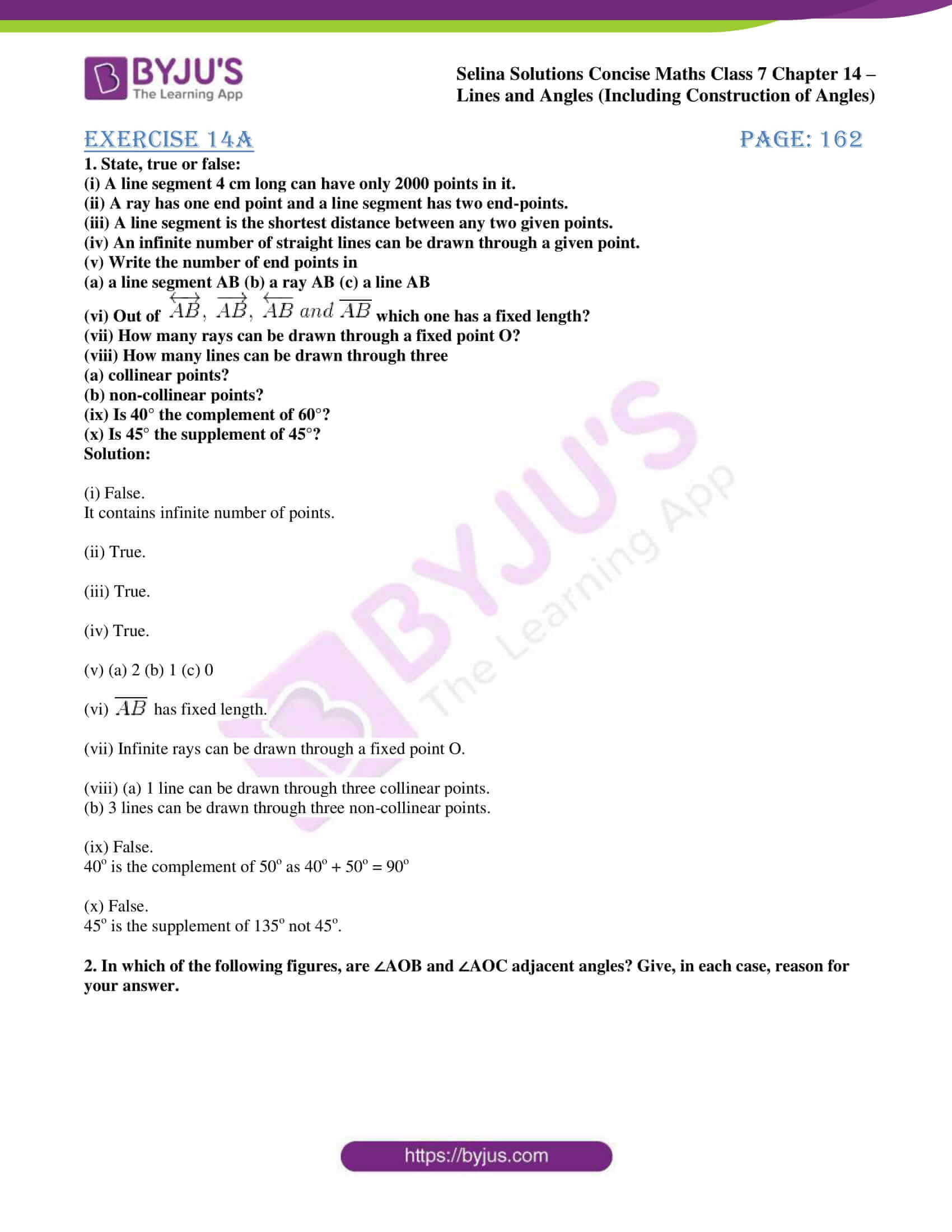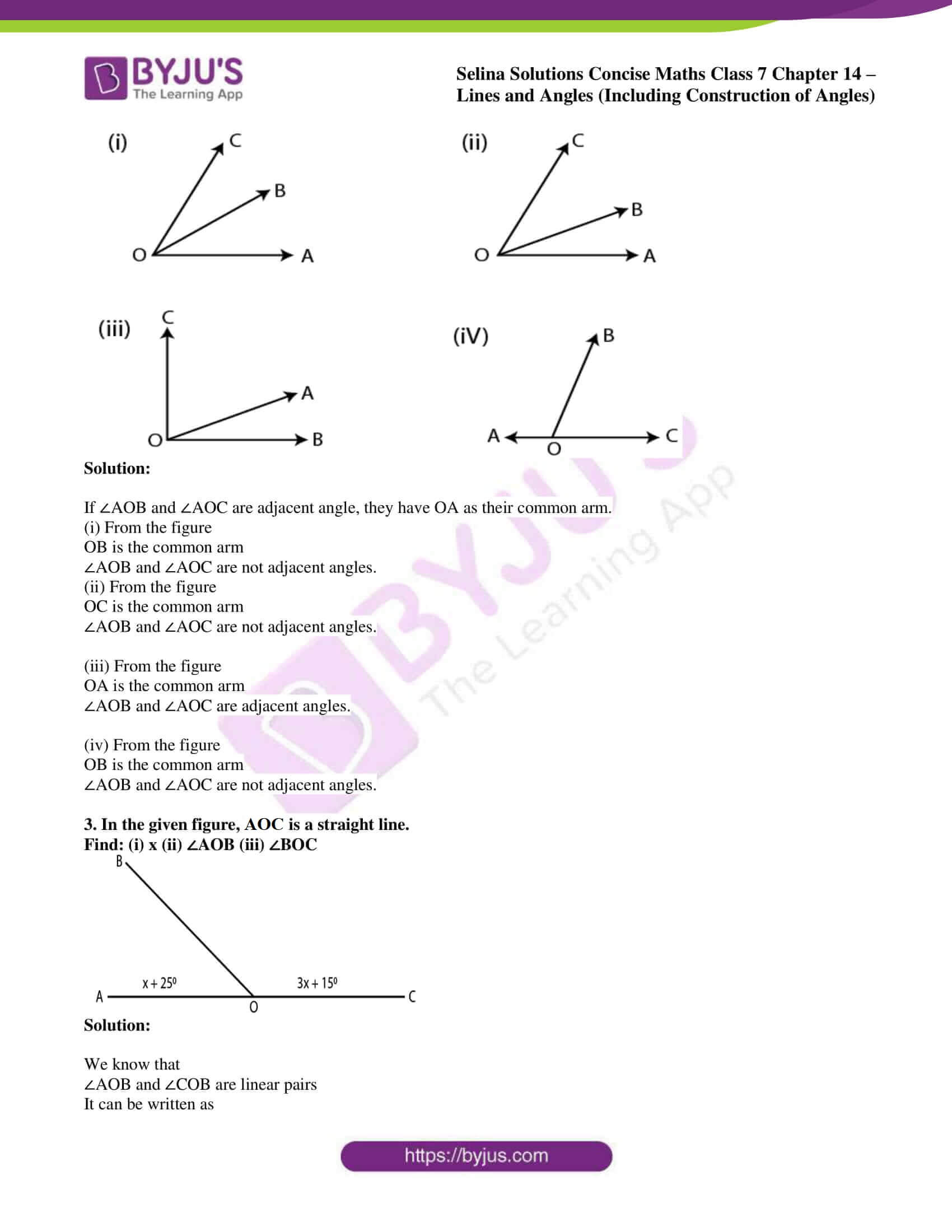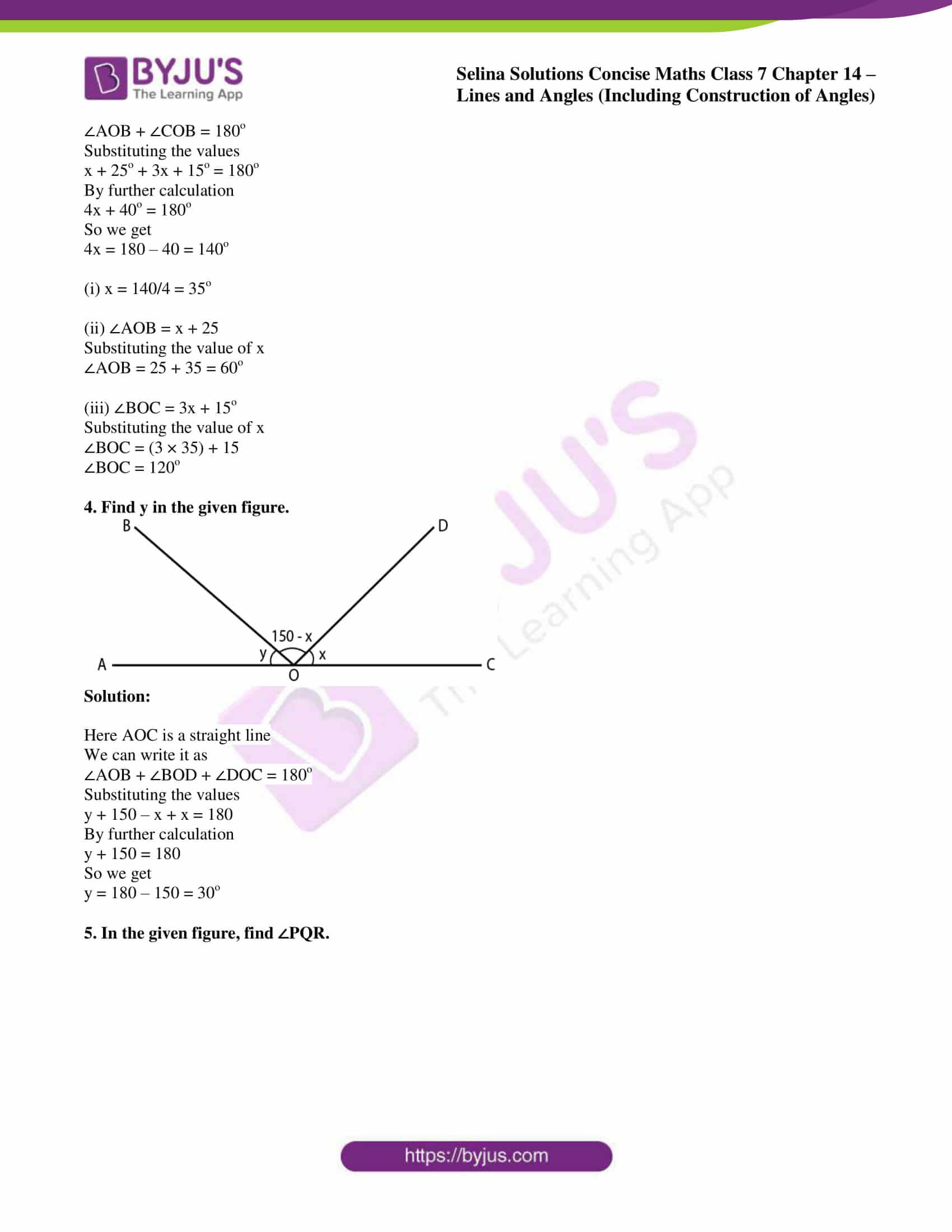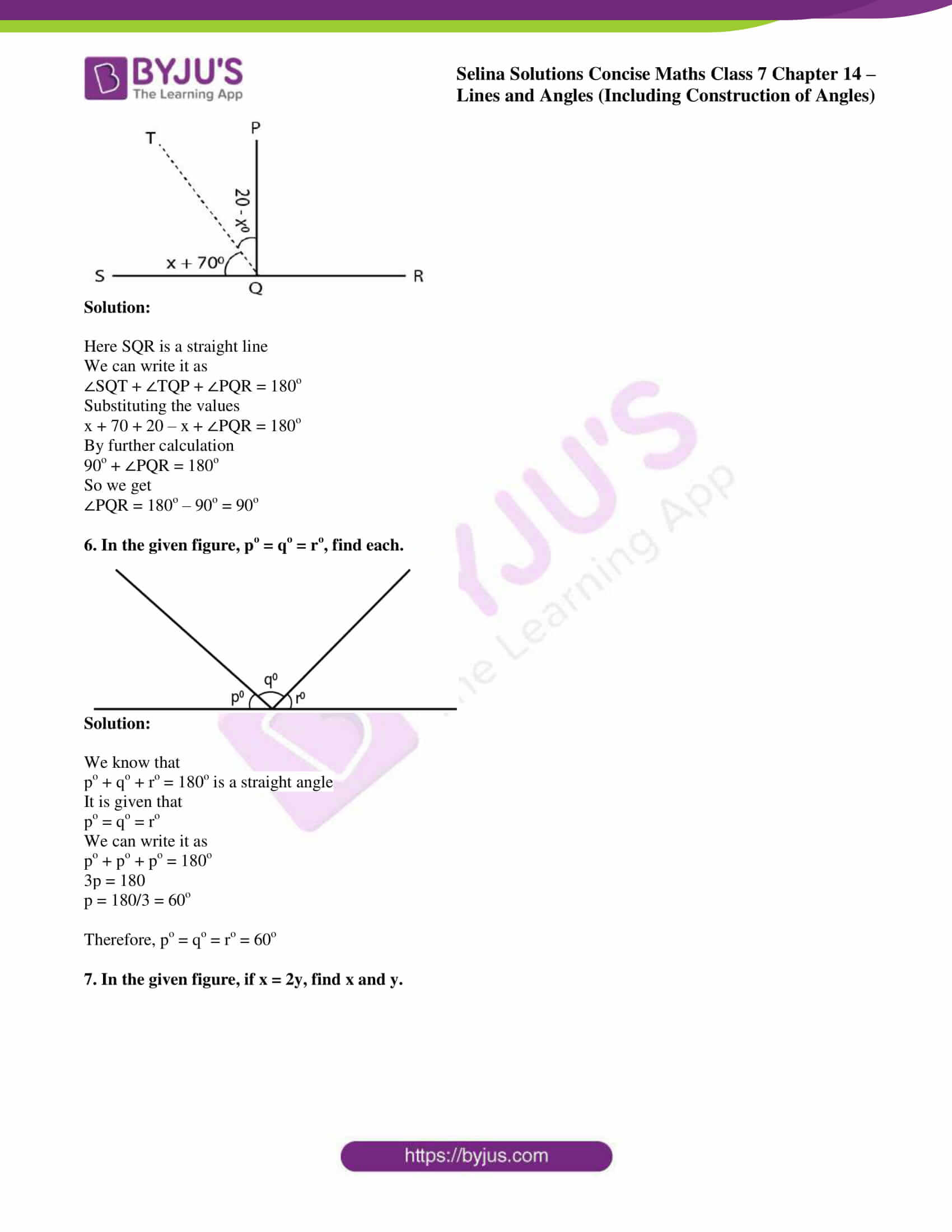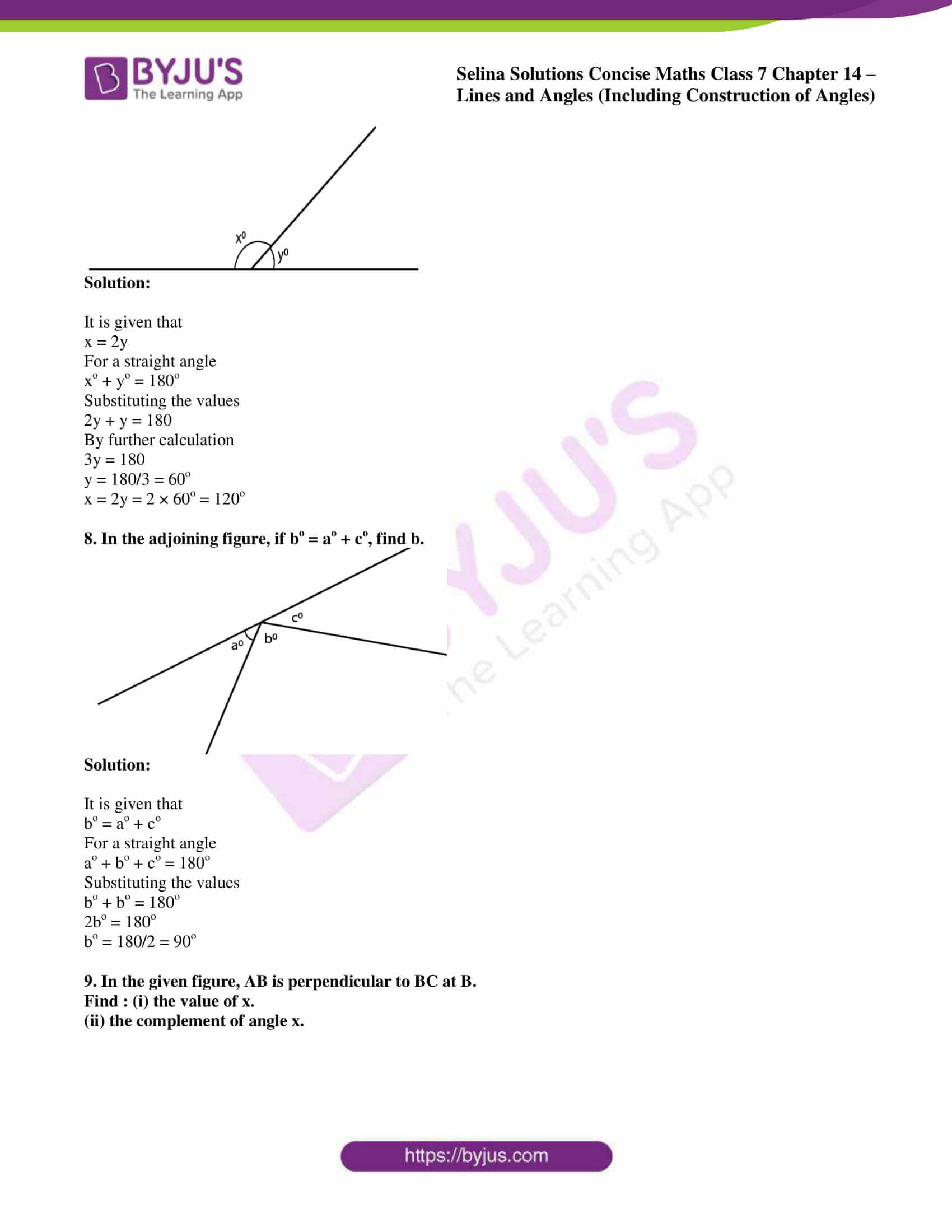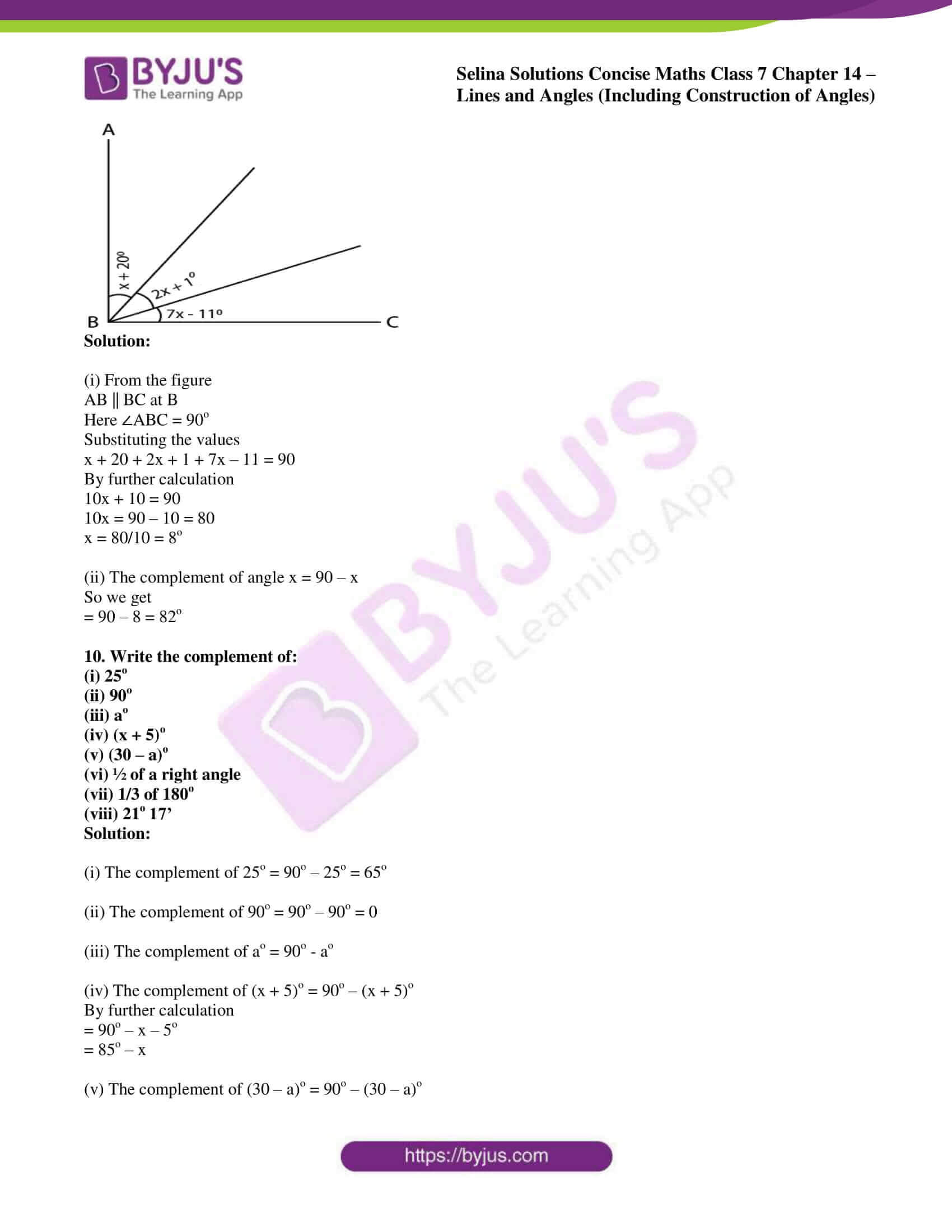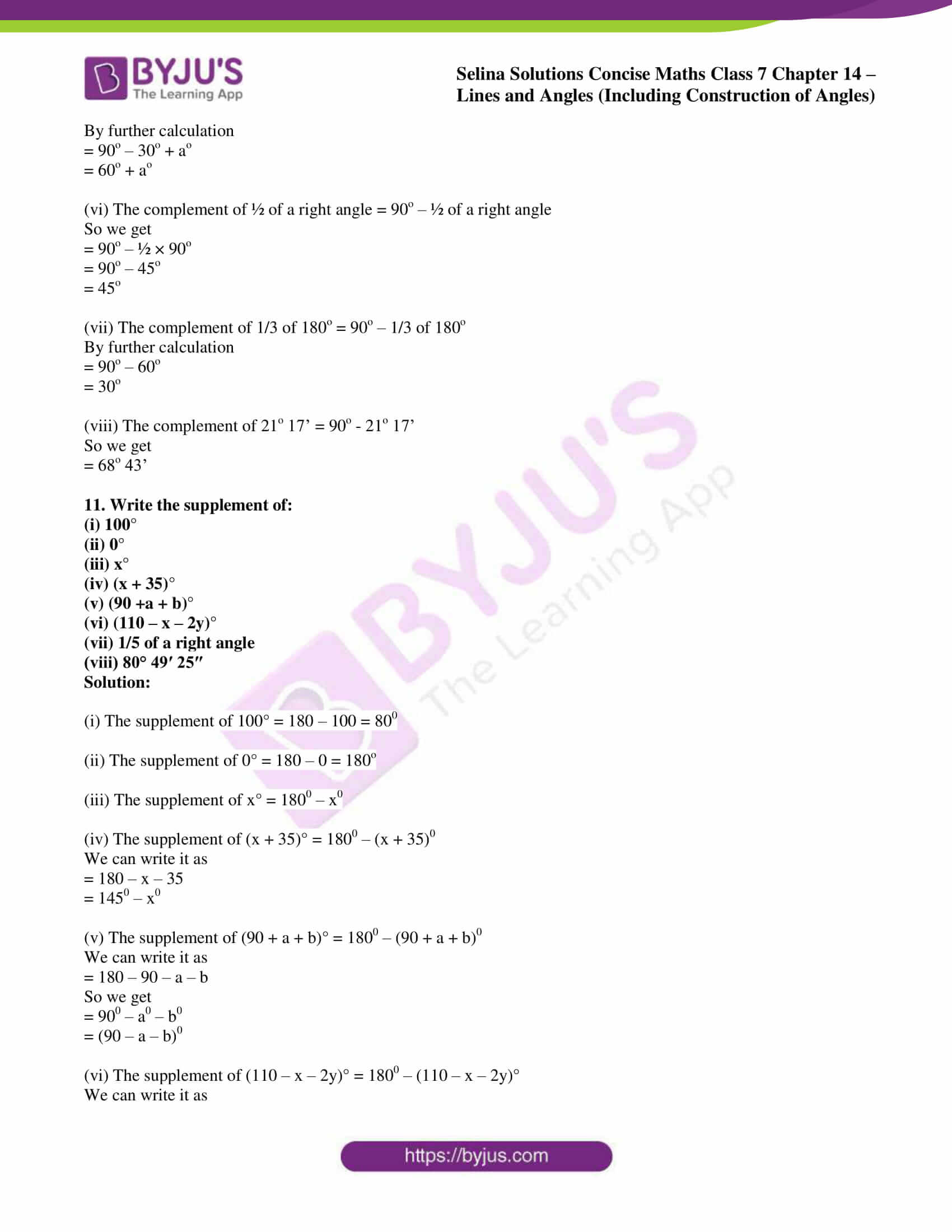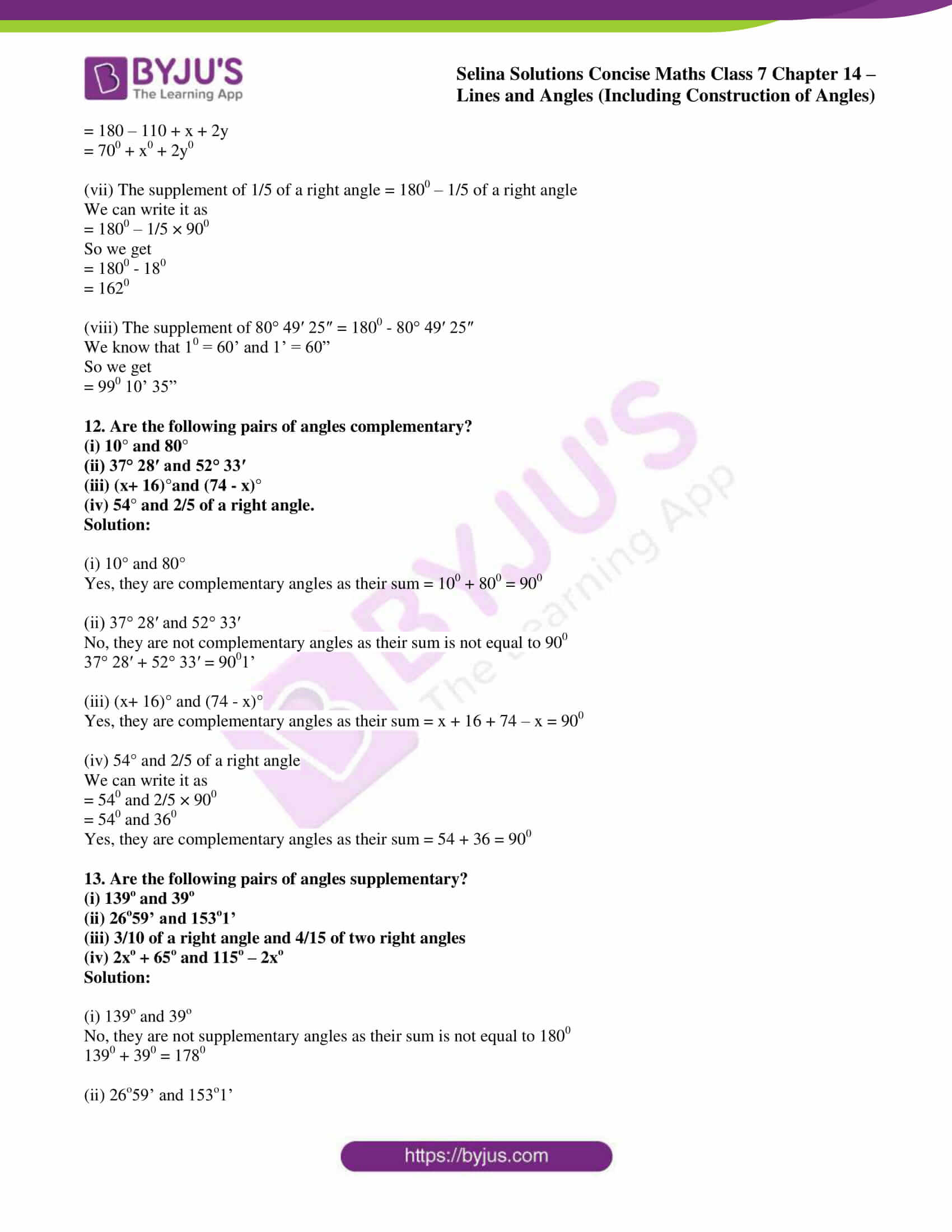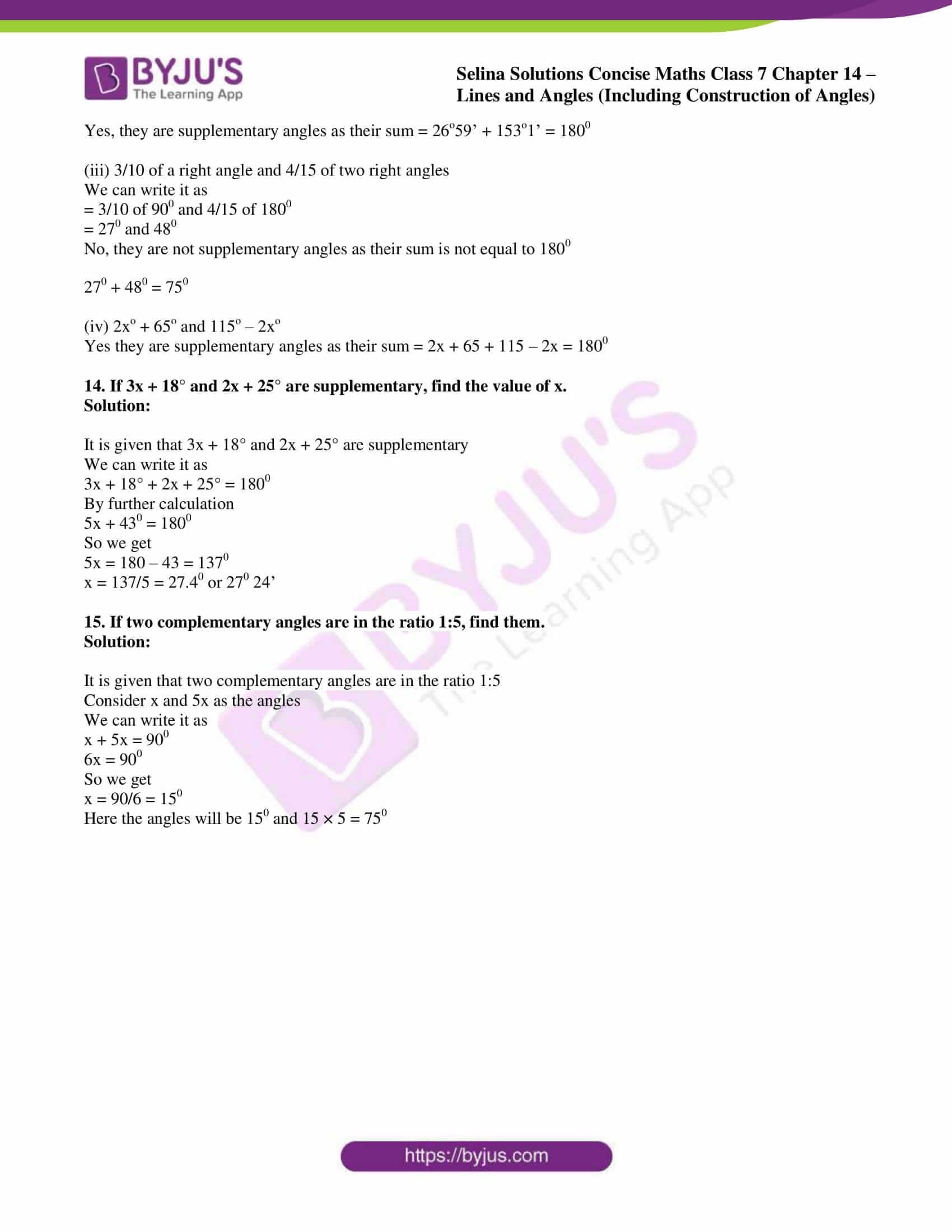### Access other exercises of Selina Solutions Concise Maths Class 7 Chapter 14: Lines and Angles (Including Construction of Angles)

Exercise 14B Solutions

Exercise 14C Solutions

### Access Selina Solutions Concise Maths Class 7 Chapter 14: Lines and Angles (Including Construction of Angles) Exercise 14A

#### Exercise 14A page: 162

1. State, true or false:

(i) A line segment 4 cm long can have only 2000 points in it.
(ii) A ray has one end point and a line segment has two end-points.
(iii) A line segment is the shortest distance between any two given points.
(iv) An infinite number of straight lines can be drawn through a given point.
(v) Write the number of end points in
(a) a line segment AB (b) a ray AB (c) a line AB
(vi) Out of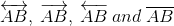which one has a fixed length?
(vii) How many rays can be drawn through a fixed point O?
(viii) How many lines can be drawn through three
(a) collinear points?
(b) non-collinear points?
(ix) Is 40° the complement of 60°?
(x) Is 45° the supplement of 45°?

Solution:

(i) False.

It contains infinite number of points.

(ii) True.

(iii) True.

(iv) True.

(v) (a) 2 (b) 1 (c) 0

(vi)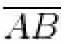has fixed length.

(vii) Infinite rays can be drawn through a fixed point O.

(viii) (a) 1 line can be drawn through three collinear points.

(b) 3 lines can be drawn through three non-collinear points.

(ix) False.

40o is the complement of 50o as 40o + 50o = 90o

(x) False.

45o is the supplement of 135o not 45o.

2. In which of the following figures, are ∠AOB and ∠AOC adjacent angles? Give, in each case, reason for your answer.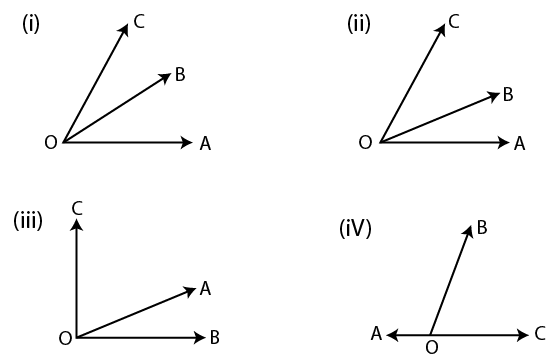Solution:

If ∠AOB and ∠AOC are adjacent angle, they have OA as their common arm.

(i) From the figure

OB is the common arm

∠AOB and ∠AOC are not adjacent angles.

(ii) From the figure

OC is the common arm

∠AOB and ∠AOC are not adjacent angles.

(iii) From the figure

OA is the common arm

∠AOB and ∠AOC are adjacent angles.

(iv) From the figure

OB is the common arm

∠AOB and ∠AOC are not adjacent angles.

3. In the given figure, AOC is a straight line.
Find: (i) x (ii) ∠AOB (iii) ∠BOC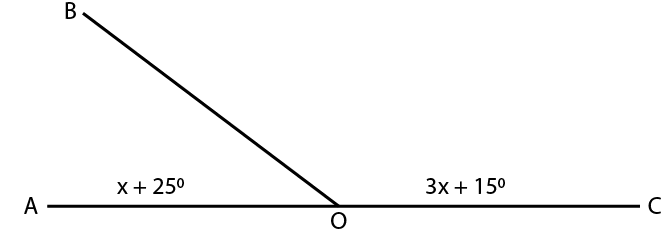Solution:

We know that

∠AOB and ∠COB are linear pairs

It can be written as

∠AOB + ∠COB = 180o

Substituting the values

x + 25o + 3x + 15o = 180o

By further calculation

4x + 40o = 180o

So we get

4x = 180 – 40 = 140o

(i) x = 140/4 = 35o

(ii) ∠AOB = x + 25

Substituting the value of x

∠AOB = 25 + 35 = 60o

(iii) ∠BOC = 3x + 15o

Substituting the value of x

∠BOC = (3 × 35) + 15

∠BOC = 120o

4. Find y in the given figure.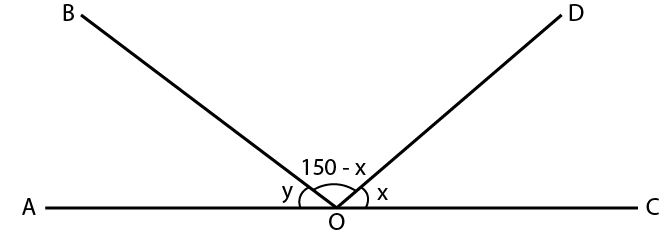Solution:

Here AOC is a straight line

We can write it as

∠AOB + ∠BOD + ∠DOC = 180o

Substituting the values

y + 150 – x + x = 180

By further calculation

y + 150 = 180

So we get

y = 180 – 150 = 30o

5. In the given figure, find ∠PQR.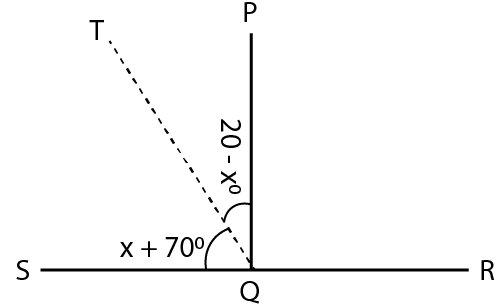Solution:

Here SQR is a straight line

We can write it as

∠SQT + ∠TQP + ∠PQR = 180o

Substituting the values

x + 70 + 20 – x + ∠PQR = 180o

By further calculation

90o + ∠PQR = 180o

So we get

∠PQR = 180o – 90o = 90o

6. In the given figure, po = qo = ro, find each.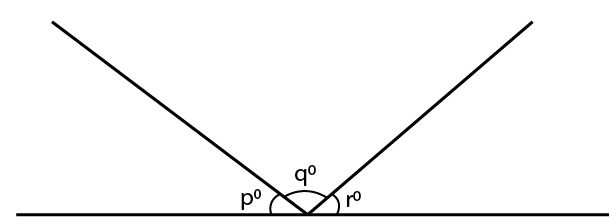Solution:

We know that

po + qo + ro = 180o is a straight angle

It is given that

po = qo = ro

We can write it as

po + po + po = 180o

3p = 180

p = 180/3 = 60o

Therefore, po = qo = ro = 60o

7. In the given figure, if x = 2y, find x and y.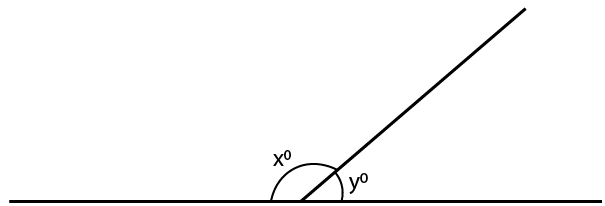Solution:

It is given that

x = 2y

For a straight angle

xo + yo = 180o

Substituting the values

2y + y = 180

By further calculation

3y = 180

y = 180/3 = 60o

x = 2y = 2 × 60o = 120o

8. In the adjoining figure, if bo = ao + co, find b.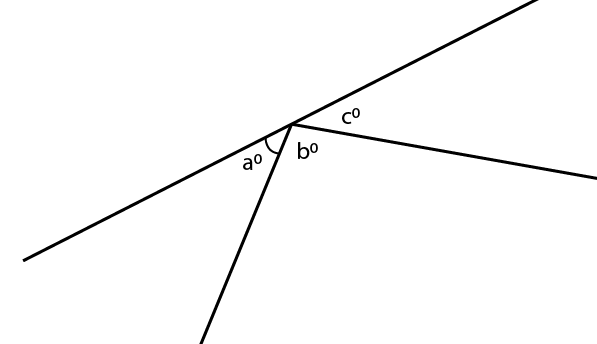Solution:

It is given that

bo = ao + co

For a straight angle

ao + bo + co = 180o

Substituting the values

bo + bo = 180o

2bo = 180o

bo = 180/2 = 90o

9. In the given figure, AB is perpendicular to BC at B.
Find : (i) the value of x.
(ii) the complement of angle x.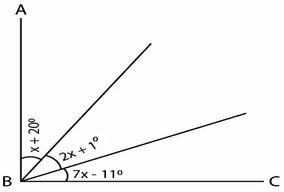Solution:

(i) From the figure

AB || BC at B

Here ∠ABC = 90o

Substituting the values

x + 20 + 2x + 1 + 7x – 11 = 90

By further calculation

10x + 10 = 90

10x = 90 – 10 = 80

x = 80/10 = 8o

(ii) The complement of angle x = 90 – x

So we get

= 90 – 8 = 82o

10. Write the complement of:

(i) 25o

(ii) 90o

(iii) ao

(iv) (x + 5)o

(v) (30 – a)o

(vi) ½ of a right angle

(vii) 1/3 of 180o

(viii) 21o 17’

Solution:

(i) The complement of 25o = 90o – 25o = 65o

(ii) The complement of 90o = 90o – 90o = 0

(iii) The complement of ao = 90o – ao

(iv) The complement of (x + 5)o = 90o – (x + 5)o

By further calculation

= 90o – x – 5o

= 85o – x

(v) The complement of (30 – a)o = 90o – (30 – a)o

By further calculation

= 90o – 30o + ao

= 60o + ao

(vi) The complement of ½ of a right angle = 90o – ½ of a right angle

So we get

= 90o – ½ × 90o

= 90o – 45o

= 45o

(vii) The complement of 1/3 of 180o = 90o – 1/3 of 180o

By further calculation

= 90o – 60o

= 30o

(viii) The complement of 21o 17’ = 90o – 21o 17’

So we get

= 68o 43’

11. Write the supplement of:

(i) 100°
(ii) 0°
(iii) x°
(iv) (x + 35)°
(v) (90 +a + b)°
(vi) (110 – x – 2y)°
(vii) 1/5 of a right angle
(viii) 80° 49′ 25″

Solution:

(i) The supplement of 100° = 180 – 100 = 800

(ii) The supplement of 0° = 180 – 0 = 180o

(iii) The supplement of x° = 1800 – x0

(iv) The supplement of (x + 35)° = 1800 – (x + 35)0

We can write it as

= 180 – x – 35

= 1450 – x0

(v) The supplement of (90 + a + b)° = 1800 – (90 + a + b)0

We can write it as

= 180 – 90 – a – b

So we get

= 900 – a0 – b0

= (90 – a – b)0

(vi) The supplement of (110 – x – 2y)° = 1800 – (110 – x – 2y)°

We can write it as

= 180 – 110 + x + 2y

= 700 + x0 + 2y0

(vii) The supplement of 1/5 of a right angle = 1800 – 1/5 of a right angle

We can write it as

= 1800 – 1/5 × 900

So we get

= 1800 – 180

= 1620

(viii) The supplement of 80° 49′ 25″ = 1800 – 80° 49′ 25″

We know that 10 = 60’ and 1’ = 60”

So we get

= 990 10’ 35”

12. Are the following pairs of angles complementary?

(i) 10° and 80°
(ii) 37° 28′ and 52° 33′
(iii) (x+ 16)°and (74 – x)°
(iv) 54° and 2/5 of a right angle.

Solution:

(i) 10° and 80°

Yes, they are complementary angles as their sum = 100 + 800 = 900

(ii) 37° 28′ and 52° 33′

No, they are not complementary angles as their sum is not equal to 900

37° 28′ + 52° 33′ = 9001’

(iii) (x+ 16)° and (74 – x)°

Yes, they are complementary angles as their sum = x + 16 + 74 – x = 900

(iv) 54° and 2/5 of a right angle

We can write it as

= 540 and 2/5 × 900

= 540 and 360

Yes, they are complementary angles as their sum = 54 + 36 = 900

13. Are the following pairs of angles supplementary?

(i) 139o and 39o

(ii) 26o59’ and 153o1’

(iii) 3/10 of a right angle and 4/15 of two right angles

(iv) 2xo + 65o and 115o – 2xo

Solution:

(i) 139o and 39o

No, they are not supplementary angles as their sum is not equal to 1800

1390 + 390 = 1780

(ii) 26o59’ and 153o1’

Yes, they are supplementary angles as their sum = 26o59’ + 153o1’ = 1800

(iii) 3/10 of a right angle and 4/15 of two right angles

We can write it as

= 3/10 of 900 and 4/15 of 1800

= 270 and 480

No, they are not supplementary angles as their sum is not equal to 1800

270 + 480 = 750

(iv) 2xo + 65o and 115o – 2xo

Yes they are supplementary angles as their sum = 2x + 65 + 115 – 2x = 1800

14. If 3x + 18° and 2x + 25° are supplementary, find the value of x.

Solution:

It is given that 3x + 18° and 2x + 25° are supplementary

We can write it as

3x + 18° + 2x + 25° = 1800

By further calculation

5x + 430 = 1800

So we get

5x = 180 – 43 = 1370

x = 137/5 = 27.40 or 270 24’

15. If two complementary angles are in the ratio 1:5, find them.

Solution:

It is given that two complementary angles are in the ratio 1:5

Consider x and 5x as the angles

We can write it as

x + 5x = 900

6x = 900

So we get

x = 90/6 = 150

Here the angles will be 150 and 15 × 5 = 750